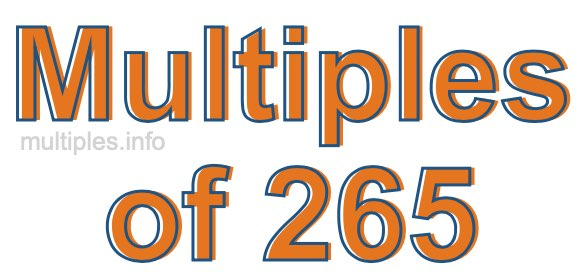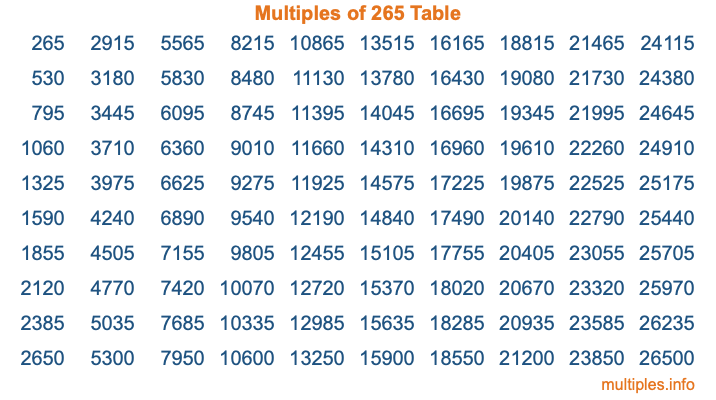Multiples of 265Welcome to the Multiples of 265 page. Here we will first teach you everything you will ever need to know about the multiples of 265, and then give you a study guide summary of everything we taught you to make sure you remember it all. Use this page to look up facts and learn information about the multiples of 265. This page will make you a multiples of two hundred sixty-five expert!

Definition of Multiples of 265
Multiples of 265 are all the numbers that when divided by 265 equal an integer. Each of the multiples of 265 are called a multiple. A multiple of 265 is created by multiplying 265 by an integer.

Therefore, to create a list of multiples of 265, you start with 1 multiplied by 265, then 2 multiplied by 265, then 3 multiplied by 265, and so on for as long as you want. Thus, the list of the first five multiples of 265 is 265, 530, 795, 1060, and 1325. To see a larger list of multiples of 265, see the printable image of Multiples of 265 further down on this page. We also have a category where you can choose any nth multiple of 265.

Multiples of 265 Checker
The Multiples of 265 Checker below checks to see if any number of your choice is a multiple of 265. In other words, it checks to see if there is any number (integer) that when multiplied by 265 will equal your number. To do that, we divide your number by 265. If the the quotient is an integer, then your number is a multiple of 265.

Is  a multiple of 265?

Least Common Multiple of 265 and ...
A Least Common Multiple (LCM) is the lowest multiple that two or more numbers have in common. This is also called the smallest common multiple or lowest common multiple and is useful to know when you are adding our subtracting fractions. Enter one or more numbers below (265 is already entered) to find the LCM.

Check out our LCM Calculator if you need more details about the Least Common Multiple or if you need the LCM for different numbers for adding and subtraction fractions.

nth Multiple of 265
As we stated above, 265 is the first multiple of 265, 530 is the second multiple of 265, 795 is the third multiple of 265, and so on. Enter a number below to find the nth multiple of 265.

th multiple of 265

Multiples of 265 vs Factors of 265
265 is a multiple of 265 and a factor of 265, but that is where the similarities end. All postive multiples of 265 are 265 or greater than 265. All positive factors of 265 are 265 or less than 265.

Below is the beginning list of multiples of 265 and the factors of 265 so you can compare:

Multiples of 265: 265, 530, 795, 1060, 1325, etc.

Factors of 265: 1, 5, 53, 265

As you can see, the multiples of 265 are all the numbers that you can divide by 265 to get a whole number. The factors of 265, on the other hand, are all the whole numbers that you can multiply by another whole number to get 265.

It's also interesting to note that if a number (x) is a factor of 265, then 265 will also be a multiple of that number (x).

Multiples of 265 vs Divisors of 265
The divisors of 265 are all the integers that 265 can be divided by evenly. Below is a list of the divisors of 265.

Divisors of 265: 1, 5, 53, 265

The interesting thing to note here is that if you take any multiple of 265 and divide it by a divisor of 265, you will see that the quotient is an integer.

Multiples of 265 Table
Below is an image of the first 100 multiples of 265 in a table. The table is in chronological order, column by column. The first column has the first ten multiples of 265, the second column has the next ten multiples of 265, and so on.The Multiples of 265 Table is also referred to as the 265 Times Table or Times Table of 265. You are welcome to print out our table for your studies.

Negative Multiples of 265
Although not often discussed or needed in math, it is worth mentioning that you can make a list of negative multiples of 265 by multiplying 265 by -1, then by -2, then by -3, and so on, to get the following list of negative multiples of 265:

-265, -530, -795, -1060, -1325, etc.

Multiples of 265 Summary
Below is a summary of important Multiples of 265 facts that we have discussed on this page. To retain the knowledge on this page, we recommend that you read through the summary and explain to yourself or a study partner why they hold true.

There are an infinite number of multiples of 265.

A multiple of 265 divided by 265 will equal a whole number.

265 divided by a factor of 265 equals a divisor of 265.

The nth multiple of 265 is n times 265.

The largest factor of 265 is equal to the first positive multiple of 265.

265 is a multiple of every factor of 265.

265 is a multiple of 265.

A multiple of 265 divided by a divisor of 265 equals an integer.

265 divided by a divisor of 265 equals a factor of 265.

Any integer times 265 will equal a multiple of 265.

Multiples of a Number
Here you can get the multiples of another number, all with the same attention to detail as we did for multiples of 265 on this page.

Multiples of
Multiples of 266
Did you find our page about multiples of two hundred sixty-five educational? Do you want more knowledge? Check out the multiples of the next number on our list!